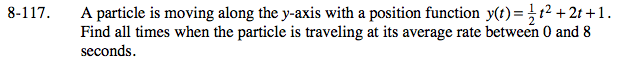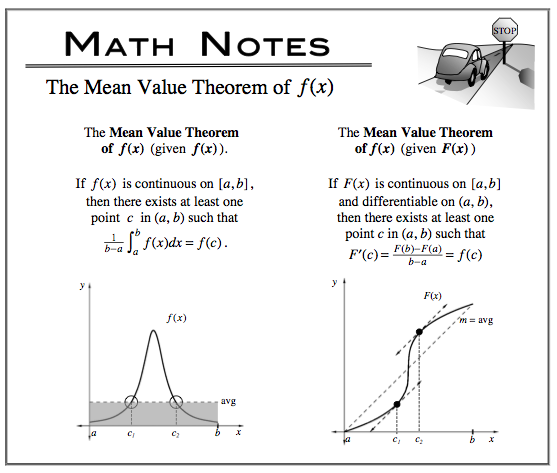### Home > CALC > Chapter 8 > Lesson 8.3.2 > Problem8-117

8-117.

A particle is moving along the y-axis with a position function y(t) =t2 + 2t + 1. Find all times when the particle is traveling at its average rate between 0 and 8 seconds. Homework Help ✎You are being asked to find all times that the actual velocity is the same as the average velocity on the interval [0, 8]. You are given the position function. How will you find the actual velocity function? How will you find the average velocity on [0,8]?

AROC = IROC somewhere

Solve for c.